### Fay’s BRR Method

Fay’s method is a modification of the BRR method, and it requires a stratified sample design with two primary sampling units (PSUs) per stratum. The total number of replicates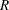is the smallest multiple of 4 that is greater than the total number of strata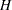. However, if you prefer a larger number of replicates, you can specify the REPS= method-option.

For each replicate, Fay’s method uses a Fay coefficient
to impose a perturbation of the original weights in the full sample that is gentler than using only half-samples, as in the traditional BRR method. The Fay coefficientcan be set by specifying the FAY =method-option. By default,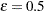if the FAY method-option is specified without providing a value for(Judkins 1990; Rao and Shao 1999). When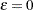, Fay’s method becomes the traditional BRR method. For more details, see Dippo, Fay, and Morganstein (1984), Fay (1984), Fay (1989), and Judkins (1990).

Letbe the number of strata. Replicates are constructed by using the firstcolumns of the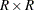Hadamard matrix, whereis the number of replicates,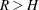. Theth (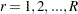) replicate is created from the full sample according to theth row of the Hadamard matrix as follows:

• If the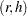th element of the Hadamard matrix is 1, then the full sample weight of the first PSU in stratumis multiplied byand the full sample weight of the second PSU is multiplied by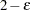to obtain theth replicate weights.

• If theth element of the Hadamard matrix is –1, then the full sample weight of the first PSU in stratumis multiplied byand the full sample weight of the second PSU is multiplied byto obtain theth replicate weights.

You can use the VARMETHOD=BRR(OUTWEIGHTS=) method-option to save the replicate weights into a SAS data set.

By default, an appropriate Hadamard matrix is generated automatically to create the replicates. You can request that the Hadamard matrix be displayed by specifying the VARMETHOD=BRR(PRINTH) method-option. If you provide a Hadamard matrix by specifying the VARMETHOD=BRR(HADAMARD=) method-option, then the replicates are generated according to the provided Hadamard matrix.

Suppose that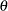is a population parameter of interest. Let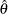be the estimate from the full sample for. Let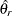be the estimate from theth replicate subsample by using replicate weights. PROC SURVEYMEANS  estimates the variance ofby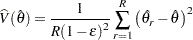withdegrees of freedom, whereis the number of strata.